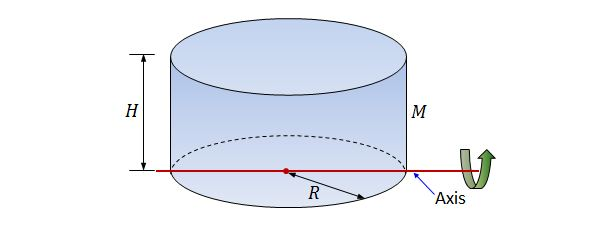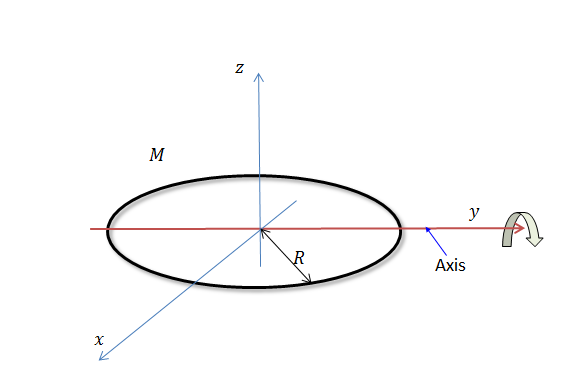Classical Mechanics

# Moment of Inertia of Mass DistributionsA disk of mass $M = 9 \text{ kg}$ and radius $R = 8 \text{ m}$ rotates about the $y$-axis, as shown in the figure above. Find its moment of inertia.Consider a solid cylinder of mass $M = 9 \text{ kg}$ with homogeneous density that has a circular base of radius $R = 5 \text{ m},$ and a height of $H = 5 \text{ m}.$ If the cylinder rotates about the diameter of the circular base, what is its moment of inertia?A ring of mass $M = 4 \text{ kg}$ and radius $R = 6 \text{ m}$ rotates about the $y$-axis, as shown in the figure above. Find its moment of inertia.

Consider a rectangular sheet of metal with width $W=2~\mbox{m}$ and length $L=5~\mbox{m}$. The sheet is in the x-y plane, with the origin right in the geometric middle of the sheet. The x-axis is parallel to the short edge, while the y-axis is parallel to the long edge. The moment of inertia about the z-axis is $I_z=10~\mbox{kg}\cdot\mbox{m}^2$ and the moment of inertia about an axis that passes diagonally through the sheet (i.e. corner to corner) in the xy plane is $I=5~\mbox{kg}\cdot\mbox{m}^2$. What is the moment of inertia about the y-axis in $\mbox{kg}\cdot\mbox{m}^2$?

Estimate the moment of inertia of a die along an axis that passes through one of the die's edges in $g~cm^2$. The mass of the die is $m=30~\mbox{g}$ and the length of each edge is $a=1~\mbox{cm}$.

Details and assumptions

Assume that the die is a perfect cube and its mass is evenly distributed.

×